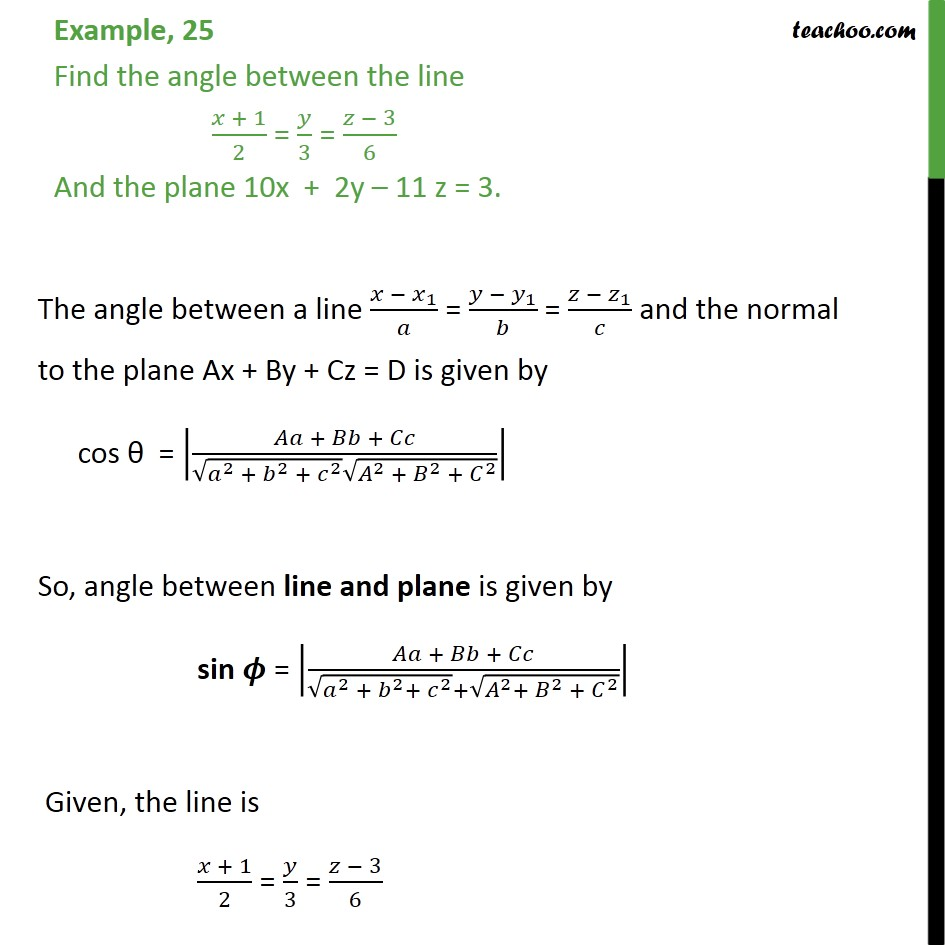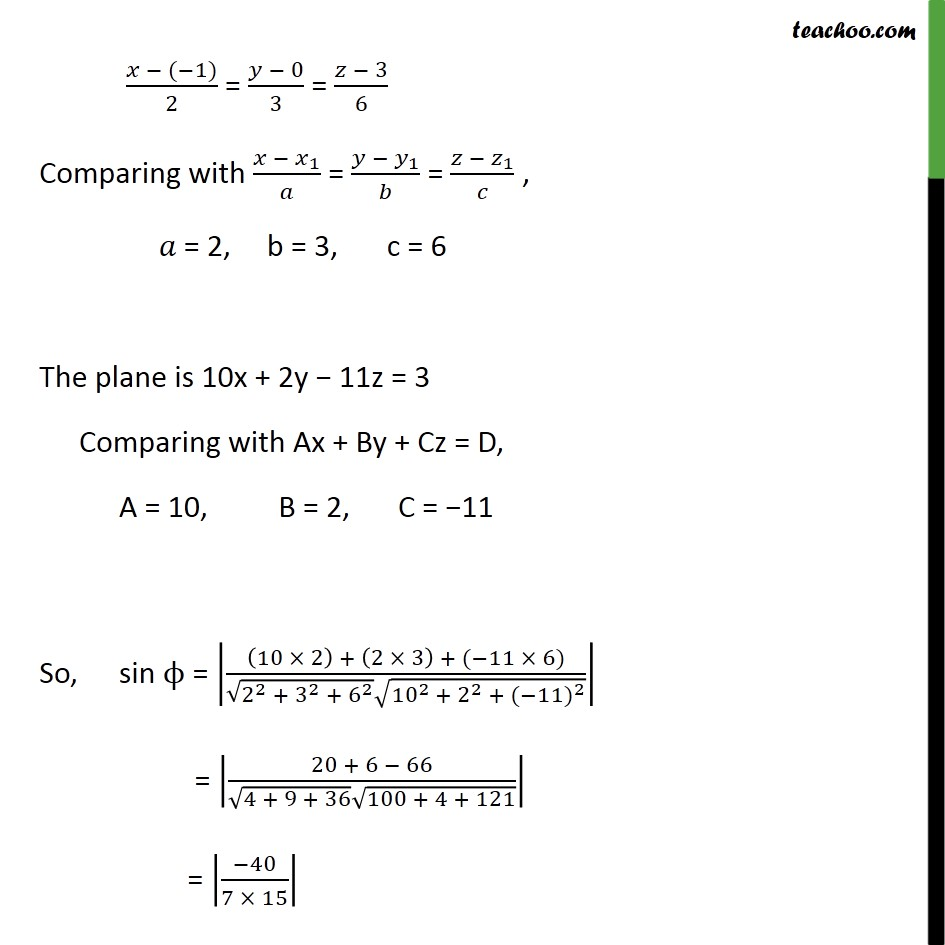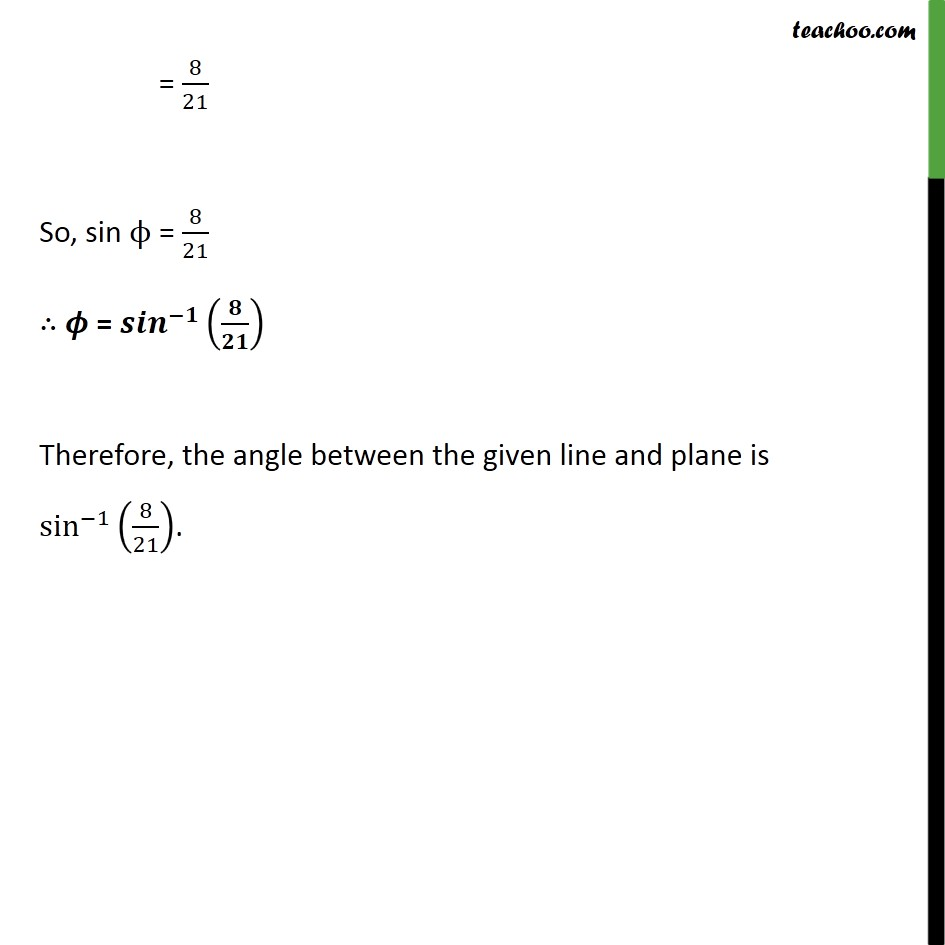1. Class 12
2. Important Question for exams Class 12
3. Chapter 11 Class 12 Three Dimensional Geometry

Transcript

Example, 25 Find the angle between the line 𝑥 + 1﷮2﷯ = 𝑦﷮3﷯ = 𝑧 − 3﷮6﷯ And the plane 10x + 2y – 11 z = 3. The angle between a line 𝑥 − 𝑥﷮1﷯﷮𝑎﷯ = 𝑦 − 𝑦﷮1﷯﷮𝑏﷯ = 𝑧 − 𝑧﷮1﷯﷮𝑐﷯ and the normal to the plane Ax + By + Cz = D is given by cos θ = 𝐴𝑎 + 𝐵𝑏 + 𝐶𝑐﷮ ﷮ 𝑎﷮2﷯ + 𝑏﷮2﷯ + 𝑐﷮2﷯﷯ ﷮ 𝐴﷮2﷯ + 𝐵﷮2﷯ + 𝐶﷮2﷯﷯﷯﷯ So, angle between line and plane is given by sin 𝝓 = 𝐴𝑎 + 𝐵𝑏 + 𝐶𝑐﷮ ﷮ 𝑎﷮2﷯ + 𝑏﷮2﷯+ 𝑐﷮2﷯﷯+ ﷮ 𝐴﷮2﷯+ 𝐵﷮2﷯ + 𝐶﷮2﷯﷯﷯﷯ Given, the line is 𝑥 + 1﷮2﷯ = 𝑦﷮3﷯ = 𝑧 − 3﷮6﷯ 𝑥 − (−1)﷮2﷯ = 𝑦 − 0﷮3﷯ = 𝑧 − 3﷮6﷯ Comparing with 𝑥 − 𝑥﷮1﷯﷮𝑎﷯ = 𝑦 − 𝑦﷮1﷯﷮𝑏﷯ = 𝑧 − 𝑧﷮1﷯﷮𝑐﷯ , 𝑎 = 2, b = 3, c = 6 The plane is 10x + 2y − 11z = 3 Comparing with Ax + By + Cz = D, A = 10, B = 2, C = −11 So, sin ϕ = 10 × 2﷯ + 2 × 3﷯ + (−11 × 6)﷮ ﷮ 2﷮2﷯ + 3﷮2﷯ + 6﷮2﷯﷯ ﷮ 10﷮2 ﷯+ 2﷮2﷯ + (−11)﷮2﷯﷯﷯﷯ = 20 + 6 − 66﷮ ﷮4 + 9 + 36﷯ ﷮100 + 4 + 121﷯﷯﷯ = −40﷮7 × 15﷯﷯ = 8﷮21﷯ So, sin ϕ = 8﷮21﷯ ∴ 𝝓 = 𝒔𝒊𝒏﷮−𝟏﷯﷮ 𝟖﷮𝟐𝟏﷯﷯﷯ Therefore, the angle between the given line and plane is sin﷮−1﷯﷮ 8﷮21﷯﷯﷯.

Chapter 11 Class 12 Three Dimensional Geometry

Class 12
Important Question for exams Class 12